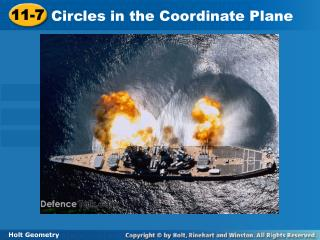DownloadDownload Presentation11-7

# 11-7

Download Presentation## 11-7

- - - - - - - - - - - - - - - - - - - - - - - - - - - E N D - - - - - - - - - - - - - - - - - - - - - - - - - - -
##### Presentation Transcript

1. 11-7 Circles in the Coordinate Plane Holt Geometry

2. Warm Up Use the Distance Formula to find the distance, to the nearest tenth, between each pair of points. 1.A(6, 2) and D(–3, –2) 2.C(4, 5) and D(0, 2) 3.V(8, 1) and W(3, 6) 4. Fill in the table of values for the equation y = x –14.

3. Objectives Write equations and graph circles in the coordinate plane. Use the equation and graph of a circle to solve problems.

4. The equation of a circle is based on the Distance Formula and the fact that all points on a circle are equidistant from the center.

5. Example 1A: Writing the Equation of a Circle Write the equation of each circle. J with center J (2, 2) and radius 4 (x – h)2 + (y – k)2 = r2 Equation of a circle Substitute 2 for h, 2 for k, and 4 for r. (x – 2)2 + (y – 2)2 = 42 (x – 2)2 + (y – 2)2 = 16 Simplify.

6. Example 1B: Writing the Equation of a Circle Write the equation of each circle. K that passes through J(6, 4) and has center K(1, –8)

7. Check It Out! Example 1a Write the equation of each circle. P with center P(0, –3) and radius 8

8. If you are given the equation of a circle, you can graph the circle by making a table or by identifying its center and radius.

9. Since the radius is , or 4, use ±4 and use the values between for x-values. Example 2A: Graphing a Circle Graph x2 + y2 = 16. Step 1 Make a table of values. Step 2 Plot the points and connect them to form a circle.

10. Example 2B: Graphing a Circle Graph (x – 3)2 + (y + 4)2 = 9.

11. (3, –4) The equation of the given circle can be written as (x – 3)2 + (y –(–4))2 = 32. So h = 3, k = –4, and r = 3. The center is (3, –4) and the radius is 3. Plot the point (3, –4). Then graph a circle having this center and radius 3.

12. Check It Out! Example 2a Graph x² + y² = 9.

13. Example 3: Radio Application An amateur radio operator wants to build a radio antenna near his home without using his house as a bracing point. He uses three poles to brace the antenna. The poles are to be inserted in the ground at three points equidistant from the antenna located at J(4, 4), K(–3, –1), and L(2, –8). What are the coordinates of the base of the antenna? Step 1 Plot the three given points. Step 2 Connect J, K, and L to form a triangle.

14. Example 3 Continued Step 3 Find a point that is equidistant from the three points by constructing the perpendicular bisectors of two of the sides of ∆JKL. The perpendicular bisectors of the sides of ∆JKL intersect at a point that is equidistant from J, K, and L. The intersection of the perpendicular bisectors is P (3, –2). P is the center of the circle that passes through J, K, and L. The base of the antenna is at P (3, –2).

15. Lesson Quiz: Part I Write the equation of each circle. 1.L with center L (–5, –6) and radius 9 2. D that passes through (–2, –1) and has center D(2, –4)

16. Lesson Quiz: Part II Graph each equation. 3.x2+ y2= 4 4. (x – 2)2 + (y + 4)2= 16

17. Lesson Quiz: Part III 5. A carpenter is planning to build a circular gazebo that requires the center of the structure to be equidistant from three support columns located at E(–2, –4), F(–2, 6), and G(10, 2). What are the coordinates for the location of the center of the gazebo?